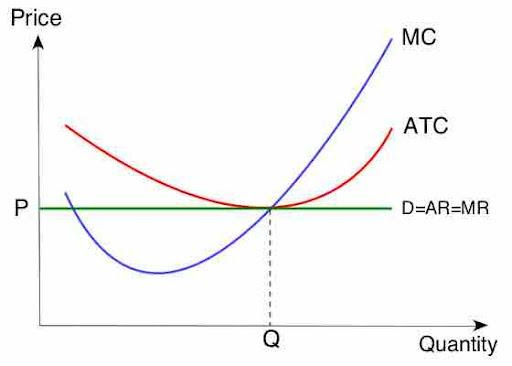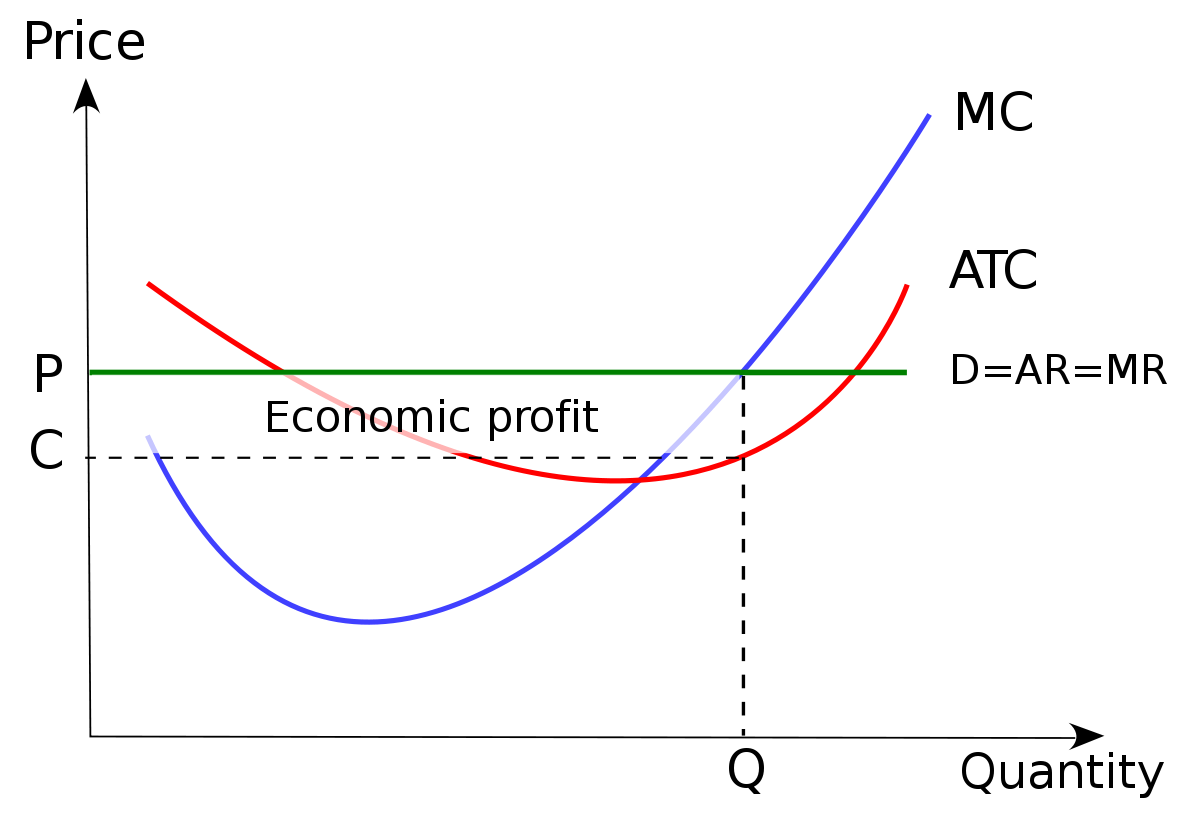Marginal profit is the profit earned by a company when they sell one more unit of production. It is calculated as the marginal revenue (i.e., the amount of revenue earned by a company from the sale of one additional item of production) minus the marginal cost (i.e., the cost of producing one more unit of production).

Marginal profit is a very important metric for firms to understand to decide how much they should produce, especially in a competitive market. However, for those SaaS enterprises using a subscription revenue model, analyzing metrics only starts here.

Baremetrics integrates directly with your payment gateways, so information about your customers is automatically piped into the Baremetrics dashboards.

You should sign up for the Baremetrics free trial, and start monitoring your subscription revenue accurately and easily.

Understanding marginal profit

To understand marginal profit, it is important to differentiate the many “profits”, as confusing the terms with “profit” in them could lead to poor decision making. Let’s look at a few of them:

• Marginal profit: Marginal profit is the amount earned by producing and selling one more unit of production.

• Average profit: Average profit is the average amount earned per unit of production.

• Total profit: Total profit is the total amount earned from selling everything produced.

Let’s take a break here for a quick example. Let’s imagine you are a clothing company using a price skimming strategy to maximize profits. You release a new t-shirt. You produce 100 t-shirts. To make these t-shirts, you need to spend \$200 for a silk screening machine and \$2 in materials and labor for each t-shirt (collectively referred to as the cost of goods sold (COGS)). You release the shirt with an initial price of \$30 and sell 30 shirts in the first month. You then lower the price to \$20 and sell 30 more. Finally, you advertise a BOGO (buy one, get one free) sale and sell the last 40 shirts for \$15 each (\$30/2).

Before showing the marginal profit, average profit, and total profit, let’s define the related revenue and cost measures and then put down some equations.

Revenue definitions:

• Marginal revenue: Marginal revenue is the amount received from selling one more unit of production.

• Average revenue: Average revenue is the average amount received per unit sold.

• Total revenue: Total revenue is the total amount received from selling everything produced.

Cost definitions:

• Marginal cost: Marginal cost is the amount spent producing one more unit of production.

• Average cost: Average cost is the average cost per unit of production.

• Total cost: Total cost is the total amount spent on everything produced.

The marginal revenue formula is:

Marginal Revenue (MR) = Change in Total Revenue/Change in Total Quantity

The marginal cost formula is:

Marginal Cost (MC) =  (Change in Costs)/(Change in Quantity)

The marginal profit formula is:

Marginal Profit (MP) = Marginal Revenue (MR) – Marginal Cost (MC)

The total profit formula is:

Total Profit (TP) = Total Revenue (TR) – Total Cost (TC)

The average profit formula is:

Average Profit = (Total Revenue – Total Cost)/Total Quantity

We now have all the tools and information needed to answer some basic questions about the example outlined above.

(1) What is the total profit?

First, let’s total the revenue. We sold 30 shirts at \$30, which is 30 × \$30 = \$900. We then sold 30 more for \$20 each, which 30 × \$20 = \$600. Finally, we sold 40 for \$15, which is 40 × \$15 = \$600. Thus, the total revenue is \$900 + \$600 + \$600 = \$2100.

Next, let’s total the expenses. There are two types of expenses here. The first is the fixed expense, which means it doesn’t change with the quantity produced. That’s the \$200 machine. The next are the variable expenses, i.e. the ones that change with volume produced, which are \$2 per shirt or \$2 × 100 = \$200 total. Thus, we have total costs of \$200 + \$200 = \$400.

Finally, total profit is the revenue minus expenses, or \$2100 – \$400 = \$1700.

(2) What is the average profit of the t-shirts?

We know that the total profit is \$1700 and the quantity produced is 100. Therefore, the average profit is \$1700/100 = \$17.

(3) What is the marginal profit of the 1st, 20th, 50th, and 100th shirts?

This will be easier to see in table format:

Shirt

Marginal Revenue

Marginal cost

MARGINAL PROFIT

1

\$30

\$202

-\$172

20

\$30

\$2

\$28

50

\$20

\$2

\$18

100

\$15

\$2

\$13

The marginal revenue of the shirts comes in three blocks. The first 30 shirts have a marginal revenue of \$30, the next 30 have a marginal revenue of \$20, and the last 40 have a marginal revenue of \$15. Marginal revenue is just the sale price.

The marginal cost of the shirts comes in two blocks. The first shirt requires buying equipment, which means it has the very high marginal cost of \$202 (the \$200 machine plus the \$2 materials and labor cost). The next 99 items all have a marginal cost of \$2 (the material and labor cost).

When you put these together, you get that the first item has a negative marginal profit of –\$172 (also called a marginal loss), then shirts 2 to 30 have the same \$28 marginal profit, 31 to 60 have a marginal profit of \$18, and finally 61 to 100 have a marginal profit of \$13.

In a SaaS business, things can often look similar. Since there can be a lot of expenses accrued before you ever sell your first subscription, the marginal profit of the first client can be negative, but since the marginal costs of running a SaaS platform (hosting fees, maintenance, etc.) are generally fairly low compared to the initial R&D the marginal profit of the following customers can be substantial.

While these calculations for a simple company selling one product can be fairly easy and intuitive, they can get pretty complicated for a SaaS business.

Baremetrics makes it easy to collect and visualize all of your sales data. When you have many clients, some are subscribed on an annual basis while others monthly, with multiple tiers and various add-ons, it can be difficult to calculate your MRR, ARR, LTV, and so much more. Thankfully, there is Baremetrics to do all of this for you.

Your SaaS company likely uses a CRM and/or payment processing software, and the data required to compute these core metrics can be all over the place. Integrating innovative software that can cull MRR values from CRM and payment processing systems is a valuable shortcut. Here too, Baremetrics can do this all for you.

Baremetrics can even monitor your SaaS quick ratio. Integrating this innovative tool can make financial analysis seamless for your SaaS company, and you can start a free trial today.

How is profit maximized when marginal profit is zero in a competitive market?

The idea that profit is maximized when marginal profit is zero sounds like voodoo. The point to remember is that total profit does not equal marginal profit, and likewise average profit does not equal marginal profit.

Marginal profit is the difference between marginal cost and marginal revenue at each point along the marginal cost and marginal revenue curves:(Note that MC is marginal cost, ATC is average total cost, D is demand, AR is average revenue, MR is marginal revenue, P is the market price, and Q is the quantity produced.)

In this figure, wherever the green line (marginal revenue, i.e. the selling price) is above the blue line (marginal cost) there is profit. If the blue line were extended to the price axis, then there’d be a marginal loss (the blue line is above the green line) for the first small quantity as the fixed costs are too high at such a low volume to turn a profit, just like our example above.

Taking all the profit in the area between the green and blue curves and subtracting the triangular area to the left where the blue curve is above the green curve would get you the total profits.

This can be represented by averaging the costs and drawing a rectangle:(Note that MC is marginal cost, ATC is average total cost, D is demand, AR is average revenue, MR is marginal revenue, P is the market price, C is cost, and Q is the quantity produced.)

In this figure, P – C (price minus average cost over that quantity) represents the average profit, while the whole rectangle is the total profits.

So why is total profit maximized when the marginal profit is zero? That’s when the rectangle is as long as possible. Even though the final item sold generates no marginal profit, each previous item has generated marginal profit, which means that selling until the marginal cost equals the marginal revenue leaves no money left on the table—you have sold to every customer from whom you can generate some profit.

Summary

Marginal profit is the amount earned on one additional item of production. It is affected by your marginal cost and marginal revenue structures.

SaaS enterprises often exhibit economies of scale, whereby their marginal cost decreases with the increase in production. In this situation, revenue can be the bigger factor in profitability.

If you’re still using spreadsheets and basic dashboards to monitor and manage your MRR, ARR, and churn, you’re not only operating inefficiently, but you’re also probably leaving money on the table.

The advanced analytics and reporting tools Baremetrics offer provide affordable, fast, and flexible means to maximize your SaaS revenue.

Our platform does all the heavy lifting for you, intelligently “automating away” meaningless numbers to uncover the true, bigger picture. The crystal-clear dashboard gives you a holistic view of your revenue, expenses, and profit for specific timeframes.